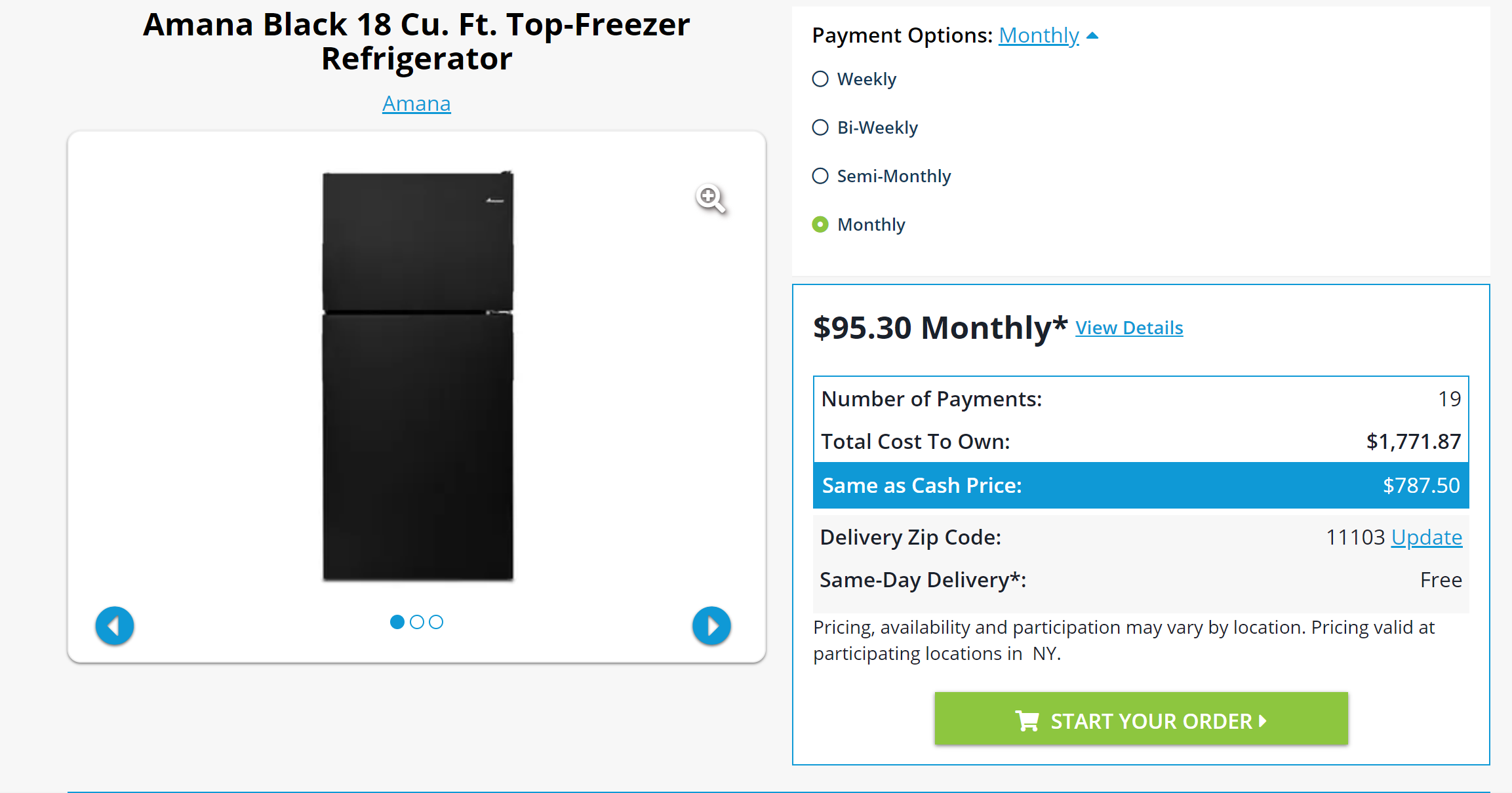CoCalc Public Filesprojects_F20 / bis_Proj8 / Interest Rate.ipynb
Author: Julia Burnside
Views : 107
Compute Environment: Ubuntu 20.04 (Default)
In [ ]:



# Determining the Interest RateRent-A-Center advertises an Amana refrigerator for $\95.30$ per month.

The actual cost of the refrigerator is $\787.50$.

The advertised payment plan cost is $\1810.70$ (disregarding taxes and promotional offer).

The annual interest rate is $122.22\%.$

You would be paying $\1023.20$ in interest.

Alternatively, it would take $9$ month of saving $\95.30$ per month in order to afford the refrigerator at the original price of $\787.50.$

In :
import math

r=1+((((95.3*19)/787.5)-1)/12)

def newton(c, d, r, n, err):
i=0
while abs(c(r)) > err and i <=n:
r=r-c(r)/d(r)
i+=1
print(r)
if i > n:
return False
else:
return r

def rate(r):
return 787.5*r**(19+1) - (787.5+95.3)*r**19 + 95.3

def rate_deriv(r):
return 787.5*(19+1)*r**19 - (787.5+95.3)*19*r**(19-1)

print('\nAnnual interest rate: ', round(12*(newton(rate, rate_deriv, r, 4, 0.0001)-1)*100, 2), '% \n')
print('Actual cost of refrigerator: $787.50 \n') print('Price paid after 19 monthly payments of$95.30: $', 19*95.3, '\n') print('Total interest paid:$', (19*95.3)-787.5, '\n')
print('Time it would take to save $95.30 per month in order to afford the refrigerator: ', math.ceil(787.5/95.3), 'months \n')  1.1026197834115743 1.1018634692604634 1.1018508808927683 Annual interest rate: 122.22 % Actual cost of refrigerator:$787.50 Price paid after 19 monthly payments of $95.30:$ 1810.7 Total interest paid: $1023.2 Time it would take to save$95.30 per month in order to afford the refrigerator: 9 months